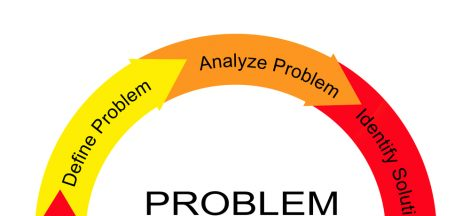Random variables are numeric description of the outcomes of an event or experiment. We had previously learnt that, an experiment is any process that results in defined outcomes. Now, these outcomes often denoted as ‘x’ is now assuming numerical descriptions. This numerical description is called RANDOM VARIABLE.

For example, the number of customers that patronized a restaurant in the first 30days of operation are as follows: 4days we had 25 customers; 10days we had 30 customers; 6days there were 35 customers and 10days we had 40 customers. In this instance, operation of restaurant is the experiment; the number of customers that patronized is the outcome – random variable (x). For these outcomes; we thus, can now get its probability function which we denote as f(x) and thus have a probability distribution.

A probability distribution of a variable is a graph, table or formula that specifies the probability associated with each possible value of a variable.

## Types of random variables

However, before we dive into probability distribution proper; it is important that we know the types of random variables we have. Basically, a random variable can either be discrete or continuous; depending on the numerical values assumed.

In the instance where numerical value of the variable is countable; or it results from counting is called Discrete Variable. A discrete variable can either be finite or infinite. Meanwhile, where the random variables assume values corresponding to any of the points contained in one or more intervals; such random variables are Continuous Variable.

So, a discrete probability distribution has discrete random variables as parameters while continuous probability distribution will have continuous random variables as its parameters.

Given the explanation above; let us now prepare the probability distribution of the example of operation of a restaurant we had earlier.

The table above is a probability distribution of the number customers that patronized the restaurant in 30 days of operation. We can also use a graph to represent it.

## Characteristics of a probability distribution:

1. The sum of all variables in a probability distribution is 1. That is to say; the sum of x is equal to 1.
2. The probabilities of the variables can not be negative. It can either be zero or greater than zero.
3. For discrete random variables, the height of the vertical lines which is the y axis, is proportional to the probability of occurrence of the variable.
4. We calculate the probability of a continuous random variable by the area under the probability distribution curve. So, we can obtain the probability or likelihood of x which is between x1 and x2 by the area under the curve between x1 and x2.

## Expected Value

After preparing the probability distribution for a random variable; we also, need to compute the mean or expected value. For a discrete random variable, the expected value (µ or Σ(x)) is the weighted average of all possible values of the random variable; where the weights are the probabilities associated with the values f(x).

The expected value of a random variable is the mean of the variables.

We still have the variance and standard deviation that we need to know how to calculate.## Navigating Business Challenges: A Comprehensive Guide to Analyzing Problems

in## Use of Communication in the Analysis of Business Problems.

in